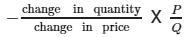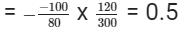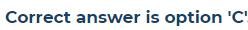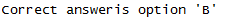Courses

# Can you explain the answer of this question below:... moreSuppose the price of movies seen at a theatre rises form Rs. 120 per person to Rs.200 per person. The theatre manager observed that the rise in prices has lead to a fall in attendance at a given movie from 300 persons to 200 persons. What is the price elasticity of demand for the movie?A: 0.5B: 0.8C: 1.00D: 1.2The answer is b. Related Test: Test: Theory Of Demand- 1

## CA Foundation Question

By Adarsh Jha · Jun 11, 2018 ·CA Foundation
Ria Chauhan answered Jul 13, 2018
The correct answer is 0.8 by using arc elasticity method .0.5 is coming by solving question with point method .

Elasticity of demand =Samitha Karnekar answered Jun 10, 2018
Arc elasticity : q1-q2 /q1+q2 × p1 +p2 /p1-p2. Where q1 =300 (0rg qty). q2=200(new qty) P1=120 (org price). P2=200 (new price)

By using arc method answer is 0.8
but in this answer is showing 0.5
if this Q will come in exam so which answer will be correct plz help

Parth Pachani answered Jun 24, 2018
Right answer is 0.8 because 200-300/200+300//200-120+200+120 100-/500//80/320 0.2/0.25 =0.8

B

Kartik Anand answered Jan 08, 2020Princy Jain answered Jun 11, 2018
Yes by using arc elasticity method 100/80*320/400

Naveen Singla answered Sep 21, 2019Suraj Chaudhary answered Apr 24, 2020
0.5

Arti Sagtani answered Oct 05, 2018
Thx

Diya Saxena answered Jan 30, 2020
Answer is b right ... by formula new demand - old demand ÷ old demand whole divided by new price - old price ÷ old price

Foxy Production answered Mar 08, 2019
All our suggestions and the exact answer for this question using arc elasticity method is 0.8( option-B )

Dilip Prajapath answered Apr 11, 2020
But sir they asked price elasticity method why we taking arc elasticity method..?

Mohammed Abbas answered Sep 28, 2019

Jalib Baba answered Feb 25, 2020
Option (a) 0.5

Arnab Chatterjee answered Dec 20, 2019
The ans should be –0.5 since price elasticity is negative

Ayush Gupta answered Jun 08, 2018
A

Shravan Desai answered Sep 07, 2019
100/80×320/500=0.8

Roy Surbhi answered Apr 24, 2020